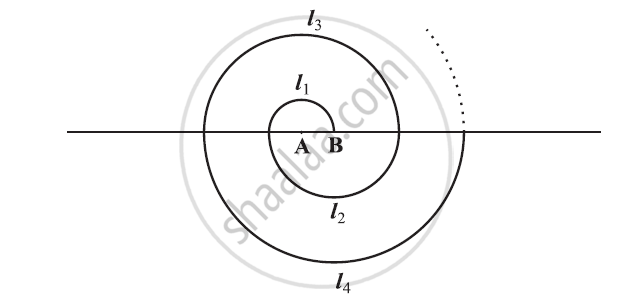# A spiral is made up of successive semicircles, with centres alternately at A and B, starting with centre at A of radii 0.5, 1.0 cm, 1.5 cm, 2.0 cm, ……… as shown in figure. What is the total length of such a spiral made up of thirteen consecutive semicircles? - Mathematics

A spiral is made up of successive semicircles, with centres alternately at A and B, starting with centre at A of radii 0.5, 1.0 cm, 1.5 cm, 2.0 cm, ……… as shown in figure. What is the total length of such a spiral made up of thirteen consecutive semicircles? (Take pi = 22/7 )#### Solution

Semi-perimeter of circle = πr

I1 = π(0.5) = pi/2

I2 = π(1) = π cm

I3 = π(1.5) = (3pi)/2

Therefore, I1, I2, I3 ,i.e. the lengths of the semi-circles are in an A.P.,

pi/2, pi, (3pi)/2, 2pi ........

a = pi/2

d = pi - pi/2=pi/2

S13 =?

We know that the sum of n terms of an a A.P. is given by

S_n = n/2[2a+(n-1)d]

= 13/2[2(pi/2)+(13-1)(pi/2)]

= 13/2[pi+(12pi)/2]

=(13/2)(7pi)

=(91pi)/2

= (91xx22)/2xx7 = 13xx11

= 143

Therefore, the length of such spiral of thirteen consecutive semi-circles will be 143 cm

Concept: Sum of First n Terms of an AP
Is there an error in this question or solution?

#### APPEARS IN

NCERT Class 10 Maths
Chapter 5 Arithmetic Progressions
Exercise 5.3 | Q 18 | Page 113